# How to Convert Time to Decimal in Excel

In this article, we need to use Convert function from Time to Decimal.

We need to convert the time figures into decimals using a spreadsheet in Excel 2016. We can manipulate time in excel 2010, 2013 and 2016 using the function CONVERT, HOUR and MINUTE.

CONVERT function is used to convert the format of the value.
Syntax:

=CONVERT(number, from_unit, to_unit)

HOUR function is used to extract the Hour value from the time figure.
Syntax:

=HOUR(serial_number)

MINUTE function is used to extract the Minute value from the time figure.
Syntax:

=MINUTE(serial_number)

Let’s get this by an example

Here we have a table, we will use the functions to convert time.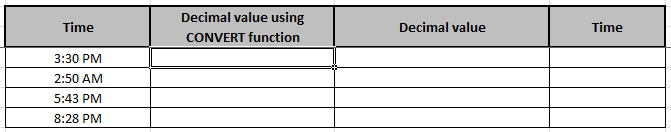Use the Formula in the selected cell and Press Enter from keyboard.
Formula:

=HOUR(B4) + CONVERT(MINUTE(B4),"mn","hr")

Explanation:
Hour function extracts the hour value from the time in B4 cell.
Minute function extracts the minute value from the time in B4 cell.
Convert function converts the extracted minute value into Hour unit.
+ sign in the formula adds both values as we want one value representing the time in decimal.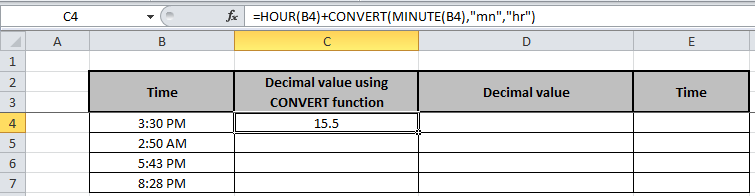Copy the formula in other cells, select the cells taking the first cell where the formula is already applied, use shortcut key Ctrl + D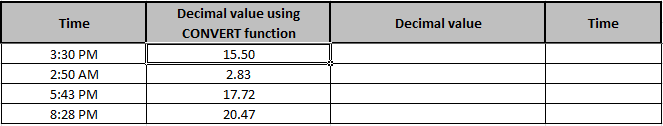We can do the same without using the Convert function.
We can convert minute to hour just be using simple logic.
As we know
60 Minutes is equal to an hour.

Minute = Hour/60

We will use this logic in the formula
Formula:

=HOUR(B4) + (MINUTE(B4)/60)

Explanation:
Hour function extracts the hour value from the time in B4 cell.
Minute function extracts the minute value from the time in B4 cell.
We divide 60 to extracted minute value to change it to Hour value.
+ sign in the formula adds both values as we want one value representing the time in decimal.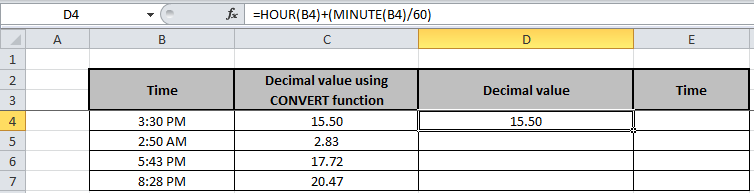Copy the formula in other cells, select the cells taking the first cell where the formula is already applied, use shortcut key Ctrl + D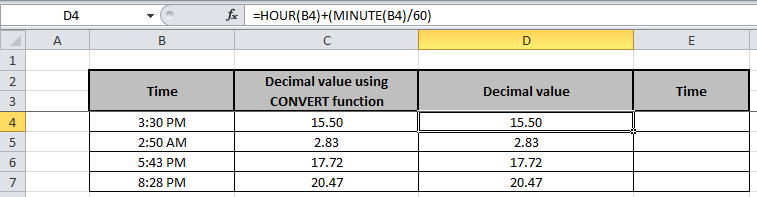As shown in the above screenshot that how we can convert time figure to decimal figure.
We can do the reverse the process simply just by using the Formula.
Formula:

=D4/24

Explanation:
The Formula takes the value from D4 cell and divides it by 24.
Choose the format cell option to change the format of the cell to see your time in time figure.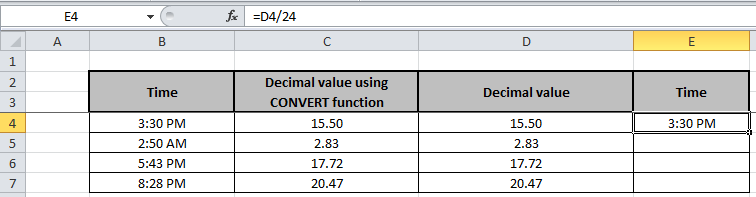Copy the formula in other cells, select the cells taking the first cell where the formula is already applied, use shortcut key Ctrl + D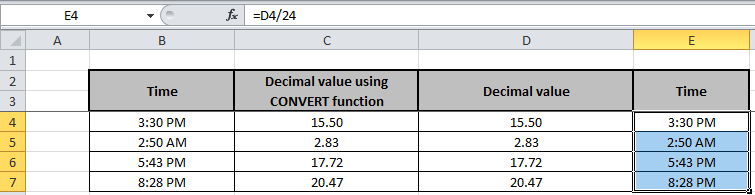See, we did it both ways. I know it's easy now. Hope you understood how to use the CONVERT function in excel. Find more articles on mathematical formulation and CONVERT function here. Please share any comment or query below in the comment box below.

Related Articles:

How to Get days between dates in Excel

Calculate years between dates in Excel

How to Add Years to a Date in Excel

Calculate Minutes Between Dates & Time In Microsoft Excel

How to Calculate hours between time in Excel

Multiplying time values and numbers in Excel

How to Calculate age from date of birth in Excel

Popular Articles:

50 Excel Shortcuts to Increase Your Productivity

How to use the VLOOKUP Function in Excel

How to use the COUNTIF function in Excel

How to use the SUMIF Function in Excel

Terms and Conditions of use

The applications/code on this site are distributed as is and without warranties or liability. In no event shall the owner of the copyrights, or the authors of the applications/code be liable for any loss of profit, any problems or any damage resulting from the use or evaluation of the applications/code.